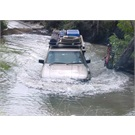# Using the 4psi Tyre Inflation Rule

### Tuesday, Feb 14, 2012 at 13:48

#### Member - Rob D (NSW)

I am not advocating the use of the 4psi rule, but a quick internet search will indicate that many people are using this rule. This blog is meant to increase the understanding of the effect of ambient temperature changes on that rule.

Care needs to be taken on how you apply the 4psi rule. The 4psi rules states that “if your tyres are inflated correctly then the tyre pressure measured when the tyres are hot (after travel) will be 4psi greater than the pressure measured when they were cold (before travel)”.

If the pressure change is greater than 4psi, then the tyre is under-inflated and the tyre pressure needs to be increased.

If the pressure change is less than 4psi then the tyre is over-inflated and the tyre pressure needs to be lowered.

This rule may be OK if the ambient air temperature is the same for both measurements, but this is usually not the case. Most people start early in the morning and then drive through the day when the ambient temperature is significantly higher than it was when the trip started.

What pressure difference does the different air temperature cause?

Fortunately there is a law of Physics known as the Gay-Lussac Law that relates the initial Pressure and Temperature to the final Pressure and Temperature for a fixed quantity of gas.

Basically it states that P1/T1 = P2/T2 for a fixed quantity of gas. Note that adding or releasing air from a tyre changes the quantity of air, even though the volume contained by the tyre is substantially the same.

This may be re-stated as P2 = P1xT2/T1

Where P1 and P2 are absolute pressure (you need to add 15psi atmospheric pressure to the tyre pressure reading, as your tyre gauge is measuring the pressure above atmospheric pressure.)

and T1 and T2 are absolute temperature (you need to add 273 degrees to the Celsius temperature reading. Minus 273 degrees Celsius is the temperature at which all molecular motion ceases.)

Example 1:
Typical northern outback temperatures in the middle of winter, when most of us travel that region are around 15C average minimum and 30C average maximum. These values will be used in this example. A cold (15C) starting pressure of 35 psi will be assumed in this example.

T1 = 15 + 273 or 288 degrees absolute
T2 = 30 + 273 or 303 degrees absolute
P1 = 35 + 15 or 50 psi absolute

P2 = 50 x 303/288 = 52.6 psi absolute

Measured pressure will be 52.6 - 15 = 37.6 psi
So the measured pressure at 15 degrees Celsius is 35psi.
and the measured pressure at 30 degrees Celsius will be 37.6psi.

or the pressure change due simply to the change in outside temperature will be 2.6 psi with no movement of the vehicle.

If the start temperature had been 10C and the mid day temperature had been 35C then:
T1 = 10 + 273 or 283 degrees absolute
T2 = 35 + 273 or 308 degrees absolute
P1 = 35 + 15 or 50 psi absolute

P2 = 50 x 308/283 = 54.4 psi absolute

Thus the pressure change due simply to the change in outside temperature will be 4.4 psi with no movement of the vehicle.

Applying the 4psi rule without allowing for ambient temperature differences will not make sense under these circumstances. People will be increasing the tyre pressure because they think they are under-inflated based on the measured pressure difference, when in-fact they may already be over-inflated.

The average change in pressure for the first example is 1 psi for a 5.8C increase in ambient temperature. The average change in pressure for the second example is 1 psi for a 5.7C rise in ambient temperature.

So as a ‘rule of thumb’ if you add 1 psi to the 4 psi rule for every 6C temperature rise in ambient temperature, you will be much closer to implementing the intention of the 4 psi rule.

For example if the ambient temperature increase between the cold and hot measurements was 18C, then you would need to add 3psi to compensate for the air temperature rise alone. In this case you would expect a 7psi pressure increase.

### TagsIf you relax at a faster pace you can get more relaxation in for a given time. Regards RobLifetime Member:My Profile  My Blog  Send Message
BlogID: 3634
Views: 15239Loading...